Publicité

# Chap06alg

Munhchimeg
16 Mar 2010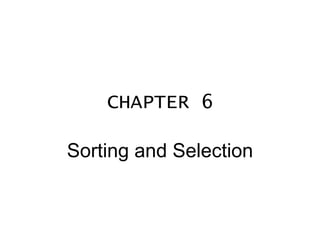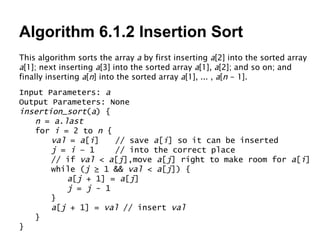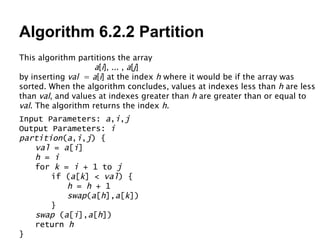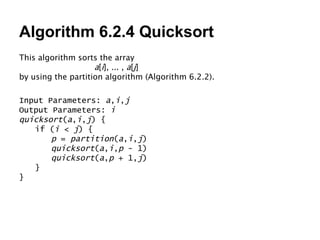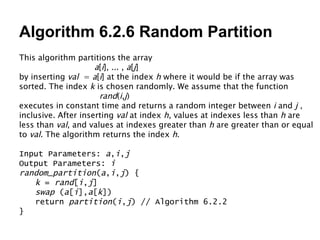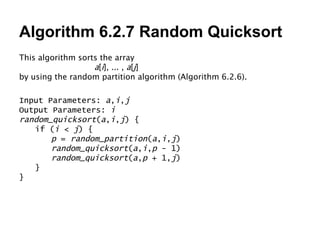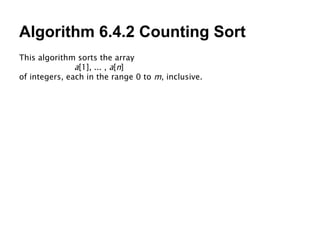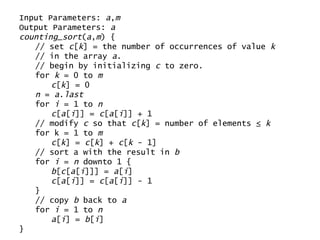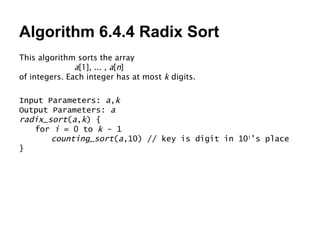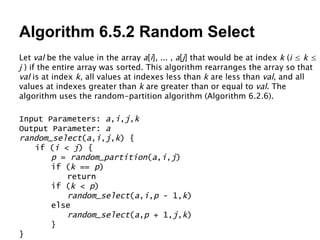1 sur 10
Publicité

### Chap06alg

1. CHAPTER 6 Sorting and Selection
2. Algorithm 6.1.2 Insertion Sort This algorithm sorts the array a by first inserting a  into the sorted array a ; next inserting a  into the sorted array a , a ; and so on; and finally inserting a [ n ] into the sorted array a , ... , a [ n - 1]. Input Parameters: a Output Parameters: None insertion_sort ( a ) { n = a . last for i = 2 to n { val = a [ i ] // save a [ i ] so it can be inserted j = i – 1 // into the correct place // if val < a [ j ],move a [ j ] right to make room for a [ i ] while ( j ≥ 1 && val < a [ j ]) { a [ j + 1] = a [ j ] j = j - 1 } a [ j + 1] = val // insert val } }
3. Algorithm 6.2.2 Partition This algorithm partitions the array a [ i ], ... , a [ j ] by inserting val = a [ i ] at the index h where it would be if the array was sorted. When the algorithm concludes, values at indexes less than h are less than val , and values at indexes greater than h are greater than or equal to val . The algorithm returns the index h . Input Parameters: a , i , j Output Parameters: i partition ( a , i , j ) { val = a [ i ] h = i for k = i + 1 to j if ( a [ k ] < val ) { h = h + 1 swap ( a [ h ], a [ k ]) } swap ( a [ i ], a [ h ]) return h }
4. Algorithm 6.2.4 Quicksort This algorithm sorts the array a [ i ], ... , a [ j ] by using the partition algorithm (Algorithm 6.2.2). Input Parameters: a , i , j Output Parameters: i quicksort ( a , i , j ) { if ( i < j ) { p = partition ( a , i , j ) quicksort ( a , i , p - 1) quicksort ( a , p + 1, j ) } }
5. Algorithm 6.2.6 Random Partition This algorithm partitions the array a [ i ], ... , a [ j ] by inserting val = a [ i ] at the index h where it would be if the array was sorted. The index k is chosen randomly. We assume that the function rand ( i , j ) executes in constant time and returns a random integer between i and j , inclusive. After inserting val at index h , values at indexes less than h are less than val , and values at indexes greater than h are greater than or equal to val . The algorithm returns the index h . Input Parameters: a , i , j Output Parameters: i random_partition ( a , i , j ) { k = rand [ i , j ] swap ( a [ i ], a [ k ]) return partition ( i , j ) // Algorithm 6.2.2 }
6. Algorithm 6.2.7 Random Quicksort This algorithm sorts the array a [ i ], ... , a [ j ] by using the random partition algorithm (Algorithm 6.2.6). Input Parameters: a , i , j Output Parameters: i random _ quicksort ( a , i , j ) { if ( i < j ) { p = random _ partition ( a , i , j ) random _ quicksort ( a , i , p - 1) random _ quicksort ( a , p + 1, j ) } }
7. Algorithm 6.4.2 Counting Sort This algorithm sorts the array a , ... , a [ n ] of integers, each in the range 0 to m , inclusive.
8. Input Parameters: a , m Output Parameters: a counting_sort ( a , m ) { // set c [ k ] = the number of occurrences of value k // in the array a . // begin by initializing c to zero. for k = 0 to m c [ k ] = 0 n = a . last for i = 1 to n c [ a [ i ]] = c [ a [ i ]] + 1 // modify c so that c [ k ] = number of elements ≤ k for k = 1 to m c [ k ] = c [ k ] + c [ k - 1] // sort a with the result in b for i = n downto 1 { b [ c [ a [ i ]]] = a [ i ] c [ a [ i ]] = c [ a [ i ]] - 1 } // copy b back to a for i = 1 to n a [ i ] = b [ i ] }
9. Algorithm 6.4.4 Radix Sort This algorithm sorts the array a , ... , a [ n ] of integers. Each integer has at most k digits. Input Parameters: a , k Output Parameters: a radix_sort ( a , k ) { for i = 0 to k - 1 counting_sort ( a ,10) // key is digit in 10 i ’s place }
10. Algorithm 6.5.2 Random Select Let val be the value in the array a [ i ], ... , a [ j ] that would be at index k ( i ≤ k ≤ j ) if the entire array was sorted. This algorithm rearranges the array so that val is at index k , all values at indexes less than k are less than val , and all values at indexes greater than k are greater than or equal to val . The algorithm uses the random-partition algorithm (Algorithm 6.2.6). Input Parameters: a , i , j , k Output Parameter: a random_select ( a , i , j , k ) { if ( i < j ) { p = random_partition ( a , i , j ) if ( k == p ) return if ( k < p ) random_select ( a , i , p - 1, k ) else random_select ( a , p + 1, j , k ) } }
Publicité